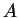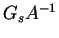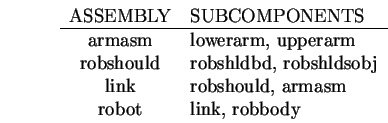Next: Network Based Geometric Reasoning Up: Estimating ASSEMBLY Reference Frames Previous: ASSEMBLY Reference Frame Calculation

### Estimating Reference Frames from Previously Recognized Subcomponents

Each previously recognized subcomponent contributes a position estimate. Suppose, the subcomponent has an estimated global reference frameand the transformation from the subcomponent to the main object is(given in the model). (If the subcomponent is connected with degrees-of-freedom, then any variables inwill be bound before this step. This is discussed here.) Then, the estimated new global frame is. Figure 9.8 illustrates how the subcomponent's reference frame relates to that of the object.In the test image, these ASSEMBLYs had their positions estimated by integrating estimates from subcomponents:The reference frame estimates for these ASSEMBLYs are summarized in Tables 9.7 and 9.8. Integrating the different position estimates sometimes gives better results and sometimes worse (e.g. robbodyside versus robot rotation). Often, there was little effect (e.g. upperarm versus armasm rotation). A key problem is that transforming the subcomponent's reference frame expands the position estimates so much that it only weakly constrained the ASSEMBLY's reference frame.

 Measured (cm) X Y Z X Estimated (cm) ASSEMBLY armasm 0.95 26.4 568. 0.60 17.1 553. robshould -13.9 17.0 558. -15.7 10.3 562. link -13.9 17.0 558. -9.7 16.3 554. robot -13.8 -32.6 564. -13.5 -35.9 562.

 Measured (rad) ROT SLANT TILT ROT Estimated (rad) ASSEMBLY armasm 3.72 2.23 2.66 3.20 2.29 3.11 robshould 0.257 2.23 6.12 0.135 2.29 6.28 link 0.257 2.23 6.12 0.055 2.29 0.05 robot 0.0 0.125 4.73 0.0 0.689 4.75

The numerical results for the whole robot in the test scene are summarized in Table 9.9. Here, the values are given in the global reference frame rather than in the camera reference frame.

Table 9.9: Measured And Estimated Spatial Parameters
PARAMETER MEASURED ESTIMATED
X 488 (cm) 486 (cm)
Y 89 (cm) 85 (cm)
Z 554 (cm) 554 (cm)